# Introduction

## Rational Numbers

Any number that can be made by dividing one integer by another. The word comes from “ratio”.

### Examples of Rational Numbers

1/2 is a rational number (1 divided by 2, or the ratio of 1 to 2)

0.75 is a rational number (3/4)

1 is a rational number (1/1)

2 is a rational number (2/1)

2.12 is a rational number (212/100)

-6.6 is a rational number (-66/10).Few more examples are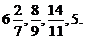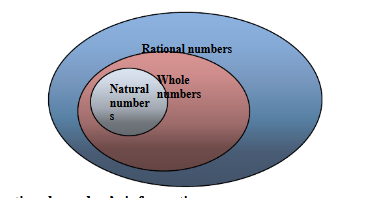### Some useful rational number’s information:

• Every natural number is a rational number but a rational number need not be a natural number.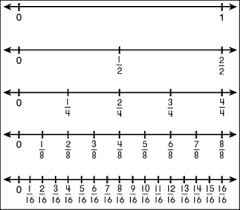e.g:. …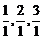etc. are natural number as well as rational numbers.

• Zero is a rational number.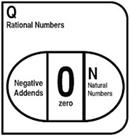(3) Every integer is a rational number but a rational number need not be an integer.

(4) Every fraction is a rational number but a rational number need not be a fraction.

(5) If   P/Q   is a rational number and m is a non-zero integer, then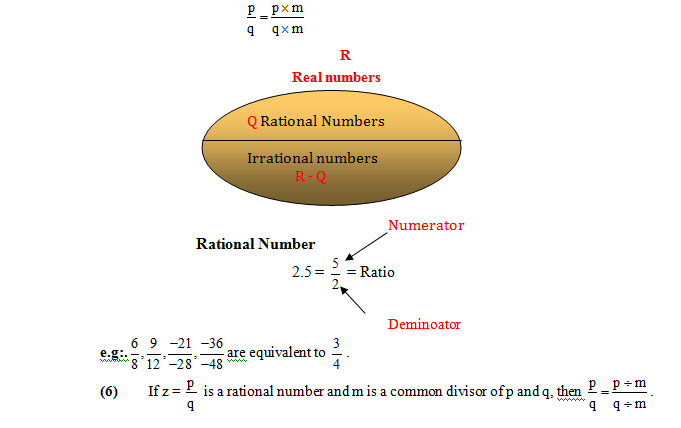To score More in your class 7 refer NCERT solutions for class 7 Maths